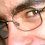## Monday, March 26, 2012

### Segmented Sieve of Eratosthenes in Java

As promised, here's a simple implementation of the segmented sieve. The segmented sieve is a very straight forward algorithm. The objective is to find all prime numbers between L and R. Typically L and R are very large (1e16-1e18), while R-L is much smaller (1e8-1e10). The odd prime numbers that have multiples in this interval are all smaller than sqrt(R) can be found by using a basic sieve. The sieve works in exactly the same way as before, except for each prime p we start sifting from its first multiple contained in the given interval. To find it, we need to find the first number n that satisfies the equation n mod p = 0. Now be q = -L mod p, then n = L + q. In fact, (L + p) mod p = (L - L) mod p = 0. L + q is the first number to be discarded; the rest of the multiples are found by repeatedly adding p to this number.
For example, if L = 100 and R = 200, all the odd primes smaller than sqrt(200) are 3, 5, 7, 11 and 13. -100 mod 3 = 2 and 102 is the first multiple of 3 contained in the interval. Likewise, -100 mod 5 = 0, -100 mod 7 = 5 and so on.
This implementation of the segmented sieve makes use of the basic sieve from my previous post. Note that for very big numbers (e.g., R=2^63, sqrt(R)>2^31) the basic sieve might not work as expected (int should be replaced by long).
`````` public class SegmentedPrimeSieve {
private final byte sieve[];
private final long start;
/**
* Creates a segmented sieve in the interval defined by the values of start
* and end.
*
* @param start
* @param end
*/
public SegmentedPrimeSieve(long start, long end) {
// if the starting value is not odd, choose the next one
start = start % 2 == 0 ? ++start : start;
// length of the byte array
int length = (int) ((end - start) / 16 + 1);
sieve = new byte[length];
// finally, let's compute the extended range
end = start + length * 16 - 2;
// find all the primes up to sqrt(end)
int maxPrime = (int) Math.floor(Math.sqrt(end));
PrimeSieve baseSieve = new PrimeSieve((int) maxPrime);
System.out.println("Sieving numbers between " + start + " and " + end);
// maximum value of k to sift multiples of primes in the form 2*k+1
int maxK = maxPrime / 2;
long intervalHalfSize = 8 * length;
// let's assume primes in the form 2*k+1 starting from k=1
for (int k = 1; k <= maxK; k++) {
// if the number is marked as a prime in the basic sieve start
// sifting all of its multiples in the given interval
if (baseSieve.get(k)) {
final int p = 2 * k + 1;
// This is the initial offset to start sifting from (-start%p)
int offset = (int) ((p - (start % p)) % p);
// if the offset is odd, start+offset is even, skip it because
// we don't have even numbers in the sieve. divide by two for
// the same reason. Note that this step is crucial!
if (offset % 2 == 1)
offset += p;
offset /= 2;
for (; offset < intervalHalfSize; offset += p) {
sieve[offset >> 3] |= (1 << (offset & 7));
}
}
}
this.start = start;
}
public boolean isPrime(long n) {
if (n < start)
throw new RuntimeException("The number " + n
+ " is too small for the values in the sieve.");
if (n == 2)
return true;
if (n == 1 || n % 2 == 0)
return false;
int dn = (int) (n - start);
int i = dn / 16;
if (i >= sieve.length)
throw new RuntimeException("The number " + n
+ " exceeds the values in the sieve.");
return ((sieve[i] >> ((dn / 2) & 7)) & 1) == 0;
}
}
``````

1.2.